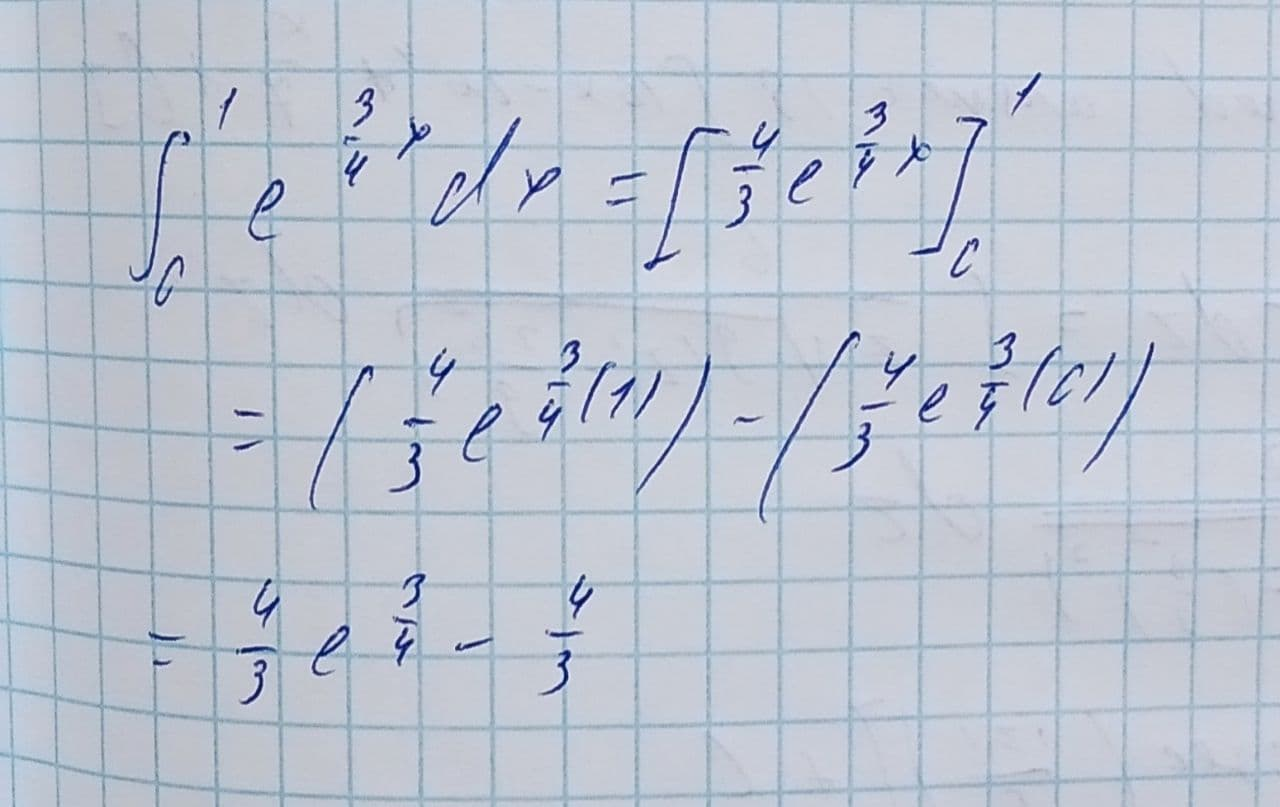Convert the indefinite integral to into a definite integral using the interval [0,1], and solve: \int_{0}^{1}e^{\frac{3}{4}x}dxTolnaio 2021-08-12 Answered
Convert the indefinite integral to into a definite integral using the interval [0,1], and solve it.
$$\displaystyle{\int_{{{0}}}^{{{1}}}}{e}^{{{\frac{{{3}}}{{{4}}}}{x}}}{\left.{d}{x}\right.}$$

• Questions are typically answered in as fast as 30 minutes

Solve your problem for the price of one coffee

• Math expert for every subject
• Pay only if we can solve itwornoutwomanC